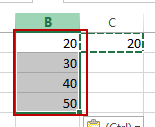# Subtract Cells

This post will guide you how to subtract a number from a range of cells in Excel. How do I subtract cells with a formula in Excel. How to subtract multiple cells from one cell or a number in Excel. How to subtract a range of cells with Paste Special feature in Excel.

Assuming that you have a list of data in range B1:B4that contain numbers and you want to subtract a number (20) from those numbers. How to achieve it. There are two methods to achieve the result.

## Method1: Subtract Cells with a Formula

You can use a formula to subtract a number from a range of cells in Excel, just type the following formula in a blank cell (C1), and then press Enter key.

`=B1-20`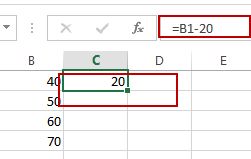Then you need to drag the AutoFill Handle from Cell C1 to C4 to apply this formula.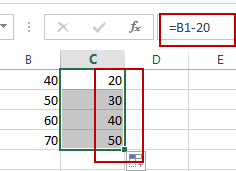## Method2: Subtract Cells with Paste Special

You can also use the Paste Special feature to subtract number 20 from a range of cells B1:B4. Just do the following steps:

#1 type the number 20 in a blank cell and then press Ctrl +C to copy it.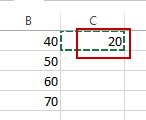#2 select the range B1:B4 that you want to subtract the number 20, right click on it, and select Paste Special menu from the popup menu list. And the Paste Special dialog will open.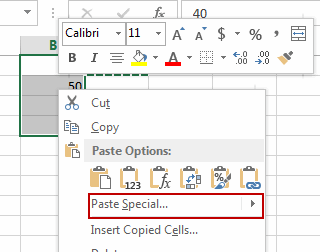#3 select subtract radio button in the Operation section, and then click OK button.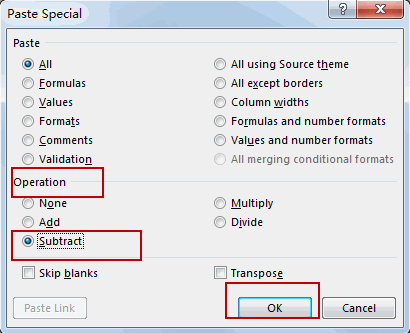#4 let’s see the result.